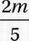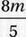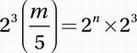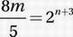# SAT Math Multiple Choice Question 747: Answer and Explanation

### Test Information

Question: 747

12. If n is a positive integer and m = 2n + 2 + 2n, what is 2n + 3 in terms of m?

• A. m
• B.• C.• D. 3m2

Explanation:

C

Notice that this question asks us to find the value of 2n +3, so we should try to solve the given equation for 2n +3.

Original equation:

m = 2n + 2 + 2n

Factor 2n from the terms on the right side:

m = 2n (22 + 1)

Simplify:

m = 2n (5)

Divide by 5:Multiply by 23 :Simplify: